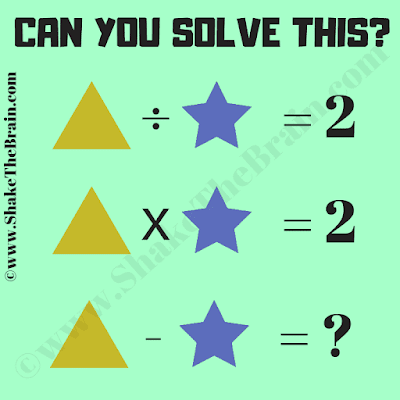This Mathematical Equations Brain Teaser is for students. In this Picture Puzzle, there are many different Mathematical equations in which variables are represented by different geometrical shapes. Can you find the values of these shape variables and then solve the last equation?Can you solve this maths brain teaser?

The answer to this "Mathematical Equations Brain Teaser for Students", can be viewed by clicking the button. Please do give your best try before looking at the answer.